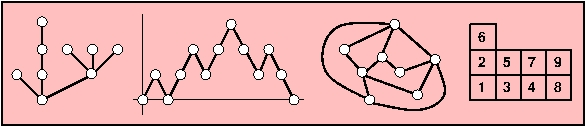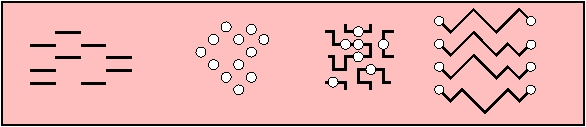Combinatorics is the study of discrete structures that appear in computer science, in mathematics, in statistical physics, in biology - in fact, they appear almost anywhere.

The most studied families are some families of trees, paths, permutations, tableaux, combinatorial maps, polynomials... a somewhat more comprehensive list is given at the end of the current page.The main activity in this group consists in obtaining theorems and other results of a mathematical nature, describing these discrete structures and their behavior. These results can be applied, among other things, to the analysis of algorithms and the study of statistical physics models.

Enumerative combinatorics

The main problems are to find decompositions for the objects, to find out how many objects exist of a given size, and to describe bijections between various families of objects.

Algebraic combinatorics

Links between combinatorics and algebra exist in both directions. Solving questions of an algebraic nature sometimes requires the enumeration of combinatorial objects. On the other hand, endowing families of combinatorial objects with some algebraic structure (what does T * T' mean when T and T' are both trees?) may help in their understanding.

Probabilistic combinatorics

What does a typical object of a given size look like? Many approaches are possible to answer this type of questions: finding efficient methods for random generation, computing limit laws for some parameters, and even proving the global convergence of discrete objects to continuous limits, after a proper renormalization.

Analysis of algorithms

Many algorithms build or explore combinatorial structures, and their analysis relies on the study of such structures. This is one reason why this topic appears in our research group. Typically, this provides average-case analysis, or analysis in distribution.

Combinatorial physics

The unfolding of new ideas in physics is often tied to the development of new combinatorial methods, and conversely some problems in combinatorics have been successfully attacked using methods inspired by statistical physics or quantum field theory.A non exhaustive list of specifically studied objects and topics

Hopf algebras, directed or undirected animals, trees (including binary search trees, simply generated trees, Galton-Watson trees, labelled trees, decorated trees, tree profiles, all with limit theorems), maps (including triangulations, quadrangulations, colored maps, hypermaps, matrix integrals and limit theorems), paths (Dyck paths, paths confined to a quarter plane, self-avoiding walks), heaps of pieces, Ewens sampling formulae, symmetric functions, Belyi functions, linear extensions, parking functions, random sampling (Boltzmann, coupling from the past), alternating sign matrices (fully packed loops, with or without symmetries), tilings, permutations (including permutation tableaux, Young tableaux, pattern avoiding permutations, permutation classes, Baxter permutations, representations of the symmetric group), polynomials (symmetric, Kerov, Jack, Tutte polynomials), abelian sandpile model, "tree-like" tableaux, watermelons.

Methods

The tools of combinatorics are varied: complex analysis, functional analysis, algebra, algebraic geometry, probability theory, random and exhaustive generation, symbolic calculus.

Recurring events

Every year we organize the Journées Combinatoire de Bordeaux.